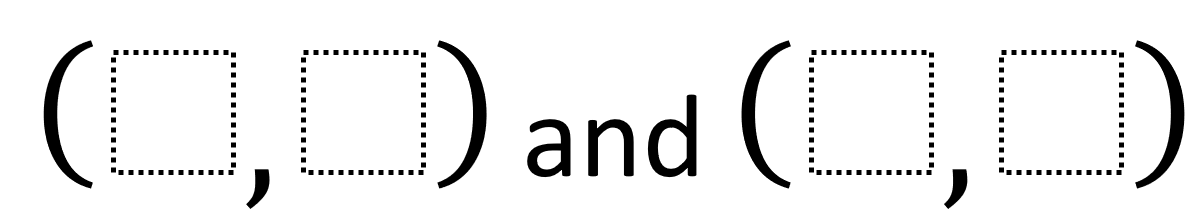# Midpoint Of A Line Segment: Longest Line Segment

Directions: Using the integers -9 to 9 at most one time each, place a digit in each box to create endpoints for the longest possible line segment whose midpoint is (1, 3).### Hint

How do you make the longest possible line segment? How do you know where its midpoint is? How might you do this algebraically or graphically? When you and another student have line segments that have a midpoint of (1, 3), how can you tell which is longer algebraically or graphically?